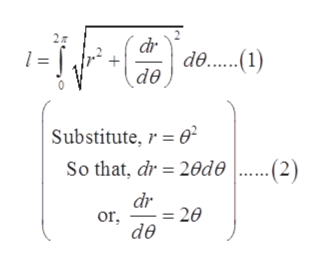# Find the exact length of the polar curver = (theta)^2from 0 <= theta <= 2(pi)

Question
6 views

Find the exact length of the polar curve

r = (theta)^2

from 0 <= theta <= 2(pi)

check_circle

Step 1

The given curve is

Step 2

To find: The exact length of given polar curve.

Step 3

The length of polar curve for the ...help_outlineImage Transcriptionclosedr de...) de |Substitute, e (2) So that, dr 20de dr 20 or de fullscreen

### Want to see the full answer?

See Solution

#### Want to see this answer and more?

Solutions are written by subject experts who are available 24/7. Questions are typically answered within 1 hour.*

See Solution
*Response times may vary by subject and question.
Tagged in

### Functions